Fractional ExponentsCustom SearchFRACTIONAL EXPONENTS Fractional exponents obey the same laws as do integral exponents. For example,Another way of expressing this would beObserve that the number 41/2 , when squared in the foregoing example, produced the number 4 as an answer. Recalling that a square root of a number N is a number x such that x2 = N, we conclude that 41/2 is equivalent to a. Thus we have a definition, as follows: A fractional exponent of the form 1/r indicates a root, the index of which is r. This is further illustrated in the following examples: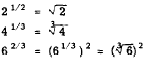Also,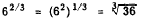Notice that in an expression such as 82/3 we can either find the cube root of 8 first or square 8 first, as shown by the following example: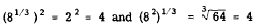All the numbers in the evaluation of 82/3 remain small if the cube root is found before raising the number to the second power. This order of operation is particularly desirable in evaluating a number like 645/6, If 64 were first raised to the fifth power, a large number would result. It would require a great deal of unnecessary effort to find the sixth root of 645. The result is obtained easily, if we writeIf an improper fraction occurs in an exponent, such as 7/3 in the expression 27/3 , it is customary to keep the fraction in that form rather than express it as a mixed number. In fraction form an exponent shows immediately what power is intended and what root is intended. However, 27/3 can be expressed in another form and simplified by changing the improper fraction to a mixed number and writing the fractional part in the radical form as follows:The law of exponents for multiplication may be combined with the rule for fractional exponents to solve problems of the following type: PROBLEM: Evaluate the expression 42.5. SOLUTION :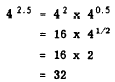Practice problems: 1. Perform the indicated division:2. Find the product: 72/5 x 71/10 x 73/10 3. Rewrite with a positive exponent and simplify: 9-1/2 4. Evaluate 1003/2 5. Evaluate (80)5 Answers:  1.2. 78/10 3.4. 1,000 5. 1 SCIENTIFIC NOTATION AND POWERS OF 10 Technicians, engineers, and others engaged in scientific work are often required to solve problems involving very large and very small numbers. Problems such asare not uncommon. Solving such problems by the rules of ordinary arithmetic is laborious and time consuming. Moreover, the tedious arithmetic process lends itself to operational errors. Also there is difficulty in locating the decimal point in the result. These difficulties can be greatly reduced by a knowledge of the powers of 10 and their use. The laws of exponents form the basis for calculation using powers of 10. The following list includes several decimals and whole numbers expressed as powers of 10:The concept of scientific notation may be demonstrated as follows: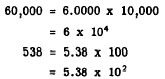Notice that the final expression in each of the foregoing examples involves a number between 1 and 10, multiplied by a power of 10. Furthermore, in each case the exponent of the power of 10 is a number equal to the number of digits between the new position of the decimal point and the original position (understood) of the decimal point. We apply this reasoning to write any number in scientific notation; that is, as a number between 1 and 10 multiplied by the appropriate power of 10. The appropriate power of 10 is found by the following mechanical steps: 1. Shift the decimal point to standard position, which is the position immediately to the right of the first nonzero digit. 2. Count the .number of digits between the new position of the decimal point and its original position. This number indicates the value of the exponent for the power of 10. 3. If the decimal point is shifted to the left, tie sign of the exponent of 10 is positive; if the decimal point is shifted to the right, the sign of the exponent is negative. The validity of this rule, for those cases in which the exponent of 10 is negative, is demonstrated as follows:Further examples of the use of scientific notation are given as follows: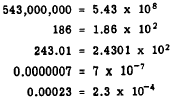Integrated Publishing, Inc. - A (SDVOSB) Service Disabled Veteran Owned Small Business